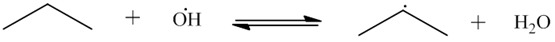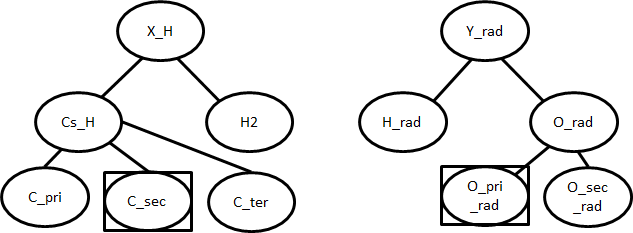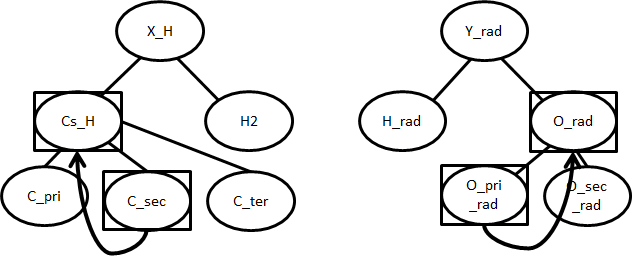# 14. Kinetics Estimation¶

This section gives in-depth descriptions of algorithms used for determining kinetic parameters. For general usage of the kinetic database see Kinetics Database.

## 14.1. Priority of Kinetic Databases¶

When multiple sources are available for kinetic parameters, the following priority is followed:

1. Seed mechanisms (based on listed order in input.py)

2. Reaction libraries (based on listed order in input.py)

3. Matched training set reactions

4. Exact template matches from rules or matched training groups (based on rank)

5. Estimated averaged rules

In the case where multiple rules or training set reactions fall under the same template node, we use a user-defined rank to determine the priority of kinetic parameters

Rank

Example methods

Rank 1

Experiment/FCI

Rank 2

W4/HEAT with very good (2-d if necessary) rotors

Rank 3

CCSD(T)-F12/cc-PVnZ with n>2 or CCSD(T)-F12/CBS with good (2-d if necessary) rotors

Rank 4

CCSD(T)-F12/DZ, with good (2-d if necessary) rotors

Rank 5

CBS-QB3 with 1-d rotors

Rank 6

Double-hybrid DFT with 1-D rotors

Rank 7

Hybrid DFT (w/ dispersion) (rotors if necessary)

Rank 8

B3LYP & lower DFT (rotors if necessary)

Rank 9

Rank 10

Direct Estimate/Guess

Rank 11

Average of Rates

Rank 0

General Estimate (Never used in generation)

The rank of 0 is assigned to kinetics that are generally default values for top level nodes that we have little faith in. It is never used in generation and its value will in fact be overriden by averages of its child nodes, which generates an averaged rate rule with rank 11.

Only non-zero rules are used in generation. A rank of 1 is assigned to the most trustworthy kinetics, while a rank of 10 is considered very poor. Thus, a rate rule of rank 3 will be given priority over a rate rule of rank 5.

Short Glossary:

FCI (Full Configuration Interaction): Exact solution to Schrodinger equation within the chosen basis set and Born-Oppenheimer approximation; possible for about 12 electrons with reasonably sized basis set (cost grows factorially with number of electrons).

Wn (Weizmann-n): Composite methods often with sub-kJ/mol accuracies; W1 is possible for about 9 heavy atoms; W1 aims to reproduce CCSD(T)/CBS; W4 aims to reproduce CCSDTQ5/CBS.

HEAT (High Accuracy Extrapolated ab inito thermochemistry): Sub-kJ/mol accuracies; essentially CCSDTQ with various corrections; similar in cost to Wn.

CBS (Complete Basis Set): Typically obtained by extrapolating to the complete basis set limit, i.e., successive cc-pVDZ, cc-pVTZ, cc-pVQZ, etc. calculations with some extrapolation formula.

CCSD(T)-F12: Coupled cluster with explicit electron correlation; chemical accuracy (1 kcal/mol) possible with double-zeta basis sets.

## 14.2. Kinetic Families¶

To show the algorithm used by kinetic families, the following H-abstraction will be used an exampleFirst the reacting atoms will be identified. Then, the familys trees will be descended as far as possible to give the reactions groups.Using the sample tree shown above, the desired template is (C_sec, O_pri_rad). The algorithm will then search the database for parameters for the template. If they are present, an exact match will be returned using the kinetics of that template. Note that an exact match refers to the nodes (C_sec, O_pri_rad) and not the molecules (propane, OH).

There may not be an entry for (C_sec, O_pri_rad) in the database. In that case, the rule will attempt to “fall up” to more general nodes:Now the preferred rule is (Cs_H, O_rad). If database contains parameters for this, those will be returned as an estimated match.

If there is still no kinetics for the template, the entire set of children for Cs_H and O_rad will be checked. For this example, this set would include every combination of {C_pri, C_sec, C_ter} with {O_pri_rad, O_sec_rad}. If any these templates have kinetics, an average of their parameters will be returned as an estimated match. The average for $$A$$ is a geometric mean, while the average for $$n$$, $$E_a$$, and $$\alpha$$ are arithmetic means.

If there are still no “sibling” kinetics, then the groups will continue to fall up to more and more general nodes. In the worst case, the root nodes may be used.

A Full List of the Kinetics Families in RMG is available.